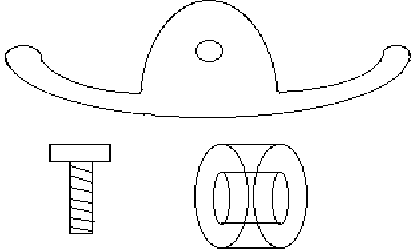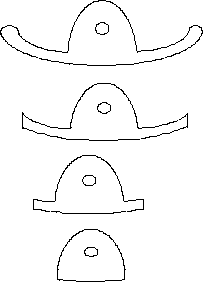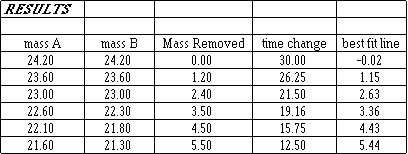## To Find the Relationship Between the Mass and the Timekeeping of the Atmos Clock

INTRODUCTION:
A torsion pendulum rotates on a horizontal plane, suspended from a suspension spring that is long, flat and thin. It rotates back and forth in a sinusoidal pattern. Because of its consistent behavior, it can be used to measure time.

The time keeping of clocks with torsion pendulums can be adjusted in four ways:
(1) by changing the strength (or thickness) of the suspension spring. A thicker spring will make the clock run faster. A thinner one will slow it down.
(2) by changing the length of the suspension spring. A longer spring will make the clock run slower. A shorter spring will make the clock run faster.
(3) by changing the mass of the pendulum. A heavier pendulum will make the clock run slower. A lighter one will make it run faster.
(4) by changing the distance away from the axis of rotation that the masses rotate. Bringing the masses closer together will make the clock run faster. Spreading the masses further apart will make the clock run slower.

Changing the mass (3) or the radius of rotation (4) affect the angular momentum, expressed by: a = m r2. When the mass or the radius are decreased, the angular momentum is decreased, and the clock runs faster. From the equation, we can see that changing the radius has a much greater effect on the angular momentum than changing the mass.

Two examples of torsion pendulum clocks are the Anniversary clock and the Jaeger Le Coultre Atmos clock. The time keeping of the Anniversary clock is adjusted by changing the strength of the suspension spring (1) and the radius of rotation (4). The time keeping of the Atmos clock is adjusted by changing the mass of the pendulum (3) or by changing the length of the suspension spring (2).

BACKGROUND:
I installed a suspension spring in an Atmos clock with a twisted spring. On a test run, it lost half an hour every 24 hours. This was too great an error to be corrected by moving the regulator (i.e. by changing the length of the spring). I was unable to buy different masses (weights) from my suppliers to meet my needs, so that I could use the table supplied by the AWI Atmos course, showing mass numbers and their effects on time keeping. My suppliers were only able to sell me two mass sizes, so I decided that there had to be another way to get this clock to keep time. I determined that I should remove five grams of mass, 2.5 g. from each side. This was done using a triple-beam scale, accurate to 0.1 gram. The clock still lost time by about twelve minutes per day, but I was able to correct this by moving the regulator almost as far as it would go.

A second Atmos clock needing a new spring came to my bench more recently. After changing the spring, it lost 28 minutes every 24 hours. I concluded that the replacement springs provided by Le Coultre must be thinner than the originals used in Atmos clocks thirty years ago. The difference between the 28 minute and 30 minute losses are relatively small, and could be accounted for in differences of spring thickness and/or pendulum mass. However, it was clear that this time more than five grams of mass would need to be removed. But how much?

METHOD:
If I removed one gram at a time, I would have at least five readings to plot a graph and draw a line or curve that I could use to approximate the mass I would need to remove. The mass removed must be equal on both sides, (unless one wants to alter the poise), so half a gram would be removed from each side in order to preserve the poise and weight distribution. Error would be minimized by removing the mass using the scale with exactly the same procedure for each side and for each reading. Each side had one round mass, two elongated masses and a screw holding them to the balance:I took mass readings of the three masses and the screw, and removed mass from one of the elongated masses, maintaining the mass distribution as much as possible:CALCULATIONS:
The graph shows that the relationship between mass removed and time gained could be assumed to be linear, (for very small changes in mass). Therefore the data can be entered into a statistical calculator and linear regression performed:

M = bT + a

a = 9.34 intercept (where T=0)

so M = -0.312T + 9.34

Linear regression is the mathematical procedure used to find the "best fit" line through a set of points on a graph like the one shown.

If the correlation coefficient were small, you would try to determine from the graph whether the relationship were linear or non-linear (polynomial) or random. If it is not linear, obviously linear regression would not apply.

The correlation coefficient for the above data is: r = -0.9986, showing that the relationship between mass removed and change in time keeping could be assumed to be linear within the range measured in this experiment for the purpose of repair. The coefficient value is negative because the gradient is negative. A perfect correlation coefficient would be ±1.

To find the change in time per gram of mass removed, we need the reciprocal of the gradient because it reflects the mass to be removed per minute of time to be gained (and since the mass decreases as the time increases, the gradient is negative). So,

T/M = 1/b = 1/-0.312 = -3.2079 = -3 min. 12.5 sec. per gram per 24 hrs.

To find the mass we need to remove, we need to find where the time change (i.e. gain or loss) is zero, the intercept, which is where T = 0, so M = 9.34 grams.

Since my scale was accurate of 0.1 g., I decided to remove mass up to 9.2 grams (or 4.6 grams from each side).

A test run revealed that the clock gained 46 seconds in 24 hours, an error small enough to correct using the regulator to lengthen the suspension spring slightly.

CONCLUSION:
The relationship between mass and time keeping could be assumed to be linear for small amounts of mass removed relative to the total mass of the pendulum, at least for the purpose of repairing Atmos clocks. Removing half a gram from each side will cause the Atmos clock to gain 3 min. 12.5 sec. per 24 hours.

CAUTION:
A change in the strength of the suspension spring will change the gradient, i.e. the time gained per gram removed. Therefore, the 3:12.5 conclusion is a guideline because of possible variations in the manufacture of Atmos suspension springs. I would urge caution in removing the full calculated mass at once: for example, instead of removing 9.3 grams as calculated, try removing 7 or 8 grams and take a reading.

As more mass is removed, the angle of swing of the pendulum, or its amplitude of oscillation, increases. Removing too much mass aggravates this unintended side effect and could cause overbanking. Take note of the angle of swing during the initial test run. Another reason to remove 7 or 8 grams instead of the full calculated amount. If the first reading you take is not approximately 28 minutes slow per 24 hours, do not use this method.

Another reason why caution should be used is that the relationship could not be assumed to be linear for larger relative masses removed. The relationship would show a curve, (where the period is proportional to the square-root of the mass).

I have used this method no fewer than five times in the last five years with consistent results. It works well for me. However, if you do not understand the physics and the maths behind this experiment, you would probably experience difficulties and should not use this method: use at your own risk!

Go to Atmos Bellows

Clock Repair Main Page
Escapements in Motion